# DAV Class 7 Maths Chapter 3 Worksheet 3 Solutions

The DAV Class 7 Maths Book Solutions Pdf and DAV Class 7 Maths Chapter 3 Worksheet 3 Solutions of Rational Numbers as Decimals offer comprehensive answers to textbook questions.

## DAV Class 7 Maths Ch 3 WS 3 Solutions

Question 1.
Express the following decimals as rational numbers in standard form.
(i) 0.25
0.25 = $$\frac{25}{100}=\frac{25 \div 25}{100 \div 25}$$
= $$\frac{1}{4}$$

(ii) -0.052
-0.052 = $$\frac{-52}{1000}=\frac{-52 \div 4}{1000 \div 4}$$
= $$\frac{-13}{250}$$

(iii) 7.50
7.50 = $$\frac{750}{100}=\frac{750 \div 50}{100 \div 50}$$
= $$\frac{15}{2}$$

(iv) – 2.15
-2.15 = $$\frac{-215}{100}=\frac{-215 \div 5}{100 \div 5}$$
= $$\frac{-43}{20}$$

(v) 0.036
0.036 = $$\frac{36}{1000}=\frac{36 \div 4}{1000 \div 4}$$
= $$\frac{9}{250}$$

(vi) -9.6
-9.6 = $$\frac{-96}{10}=\frac{-96 \div 2}{10 \div 2}$$
= $$\frac{-48}{5}$$

(vii) 31.25
31.25 = $$\frac{3125}{100}=\frac{3125 \div 25}{100 \div 25}$$
= $$\frac{125}{4}$$

(viii) 16.32
16.32 = $$\frac{1632}{100}=\frac{1632 \div 4}{100 \div 4}$$
= $$\frac{408}{25}$$

(ix) 0.107
0.107 = $$\frac{107}{1000}$$ (standard form)

Question 2.
(i) 2.5, 7.51 and 11.501
2.5 + 7.51 + 11.501
= 2.500 + 7.510 + 11.501
= 21.511
OR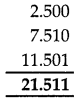(ii) 12, 5.96 and 3.076
12 + 5.96 + 3.076
= 12.000 + 5.960 + 3.076
= 21.036
OR(iii) 9.08, 19.76 and 20.54
9.08 + 19.76 + 20.54
= 49.38
OR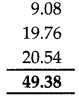(iv) 3.009, 0.592 and 14.745
3.009 + 0.592 + 14.745
= 18.346
OR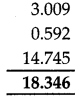(v) 19, 9.5, 12.06 and 17.921
19 + 9.5 + 12.06 + 17.921
= 19.000 + 9.500 + 12.060 + 17.921
= 58.481
ORQuestion 3.
Compute the following products of decimals.
(i) 2.9 × 3.5
2.9 × 3.5 = 29 × 35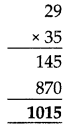Now putting the decimal in the result, we get 10.15

(ii) 37 × 12.76
37 × 12.76 = 1276 × 37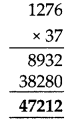Now putting the decimal in the result, we get 472.12

(iii) 0.84 × 8.8
(iii) 0.84 × 8.8 = 84 × 88 84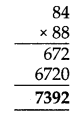Now putting the decimal in the result, we get 7.392

(iv) 2.56 × 11.09
2.56 × 11.09 = 256 × 1109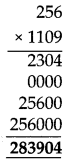Putting the decimal in the result, we get 28.3904

(v) 12.4 × 15.7 × 13.2
12.4 × 15.7 = 124 × 157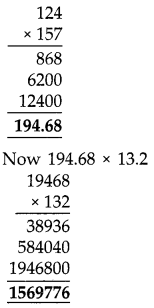Puttting the decimal = 1569.776

Question 4.
(i) 59.049 ÷ 9(ii) 6.4 ÷ 0.2(iii) 0.015 ÷ 3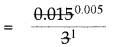= 0.005

(iv) 0.014 ÷ 12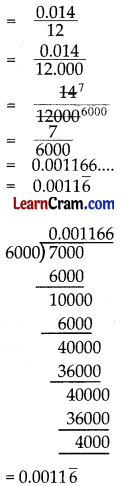(v) 0.02472 ÷ 0.008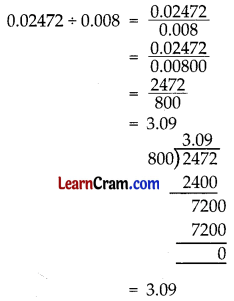(vi) 51.51 ÷ 0.17Question 5.
Evaluate the following:
(i) 25.75 + 2.09 – 13.6
25.75 + 2.09 – 13.6
= (25.75 + 2.09) – 13.60
= 27.84 – 13.60
= 14.24

(ii) 37 -16.58 + 12.25
37-16.58 + 12.25
= 37.00 – 16.58 + 12.25
= (37.00 + 12.25) – 16.58
= 49.25 – 16.58
= 32.67

(iii) 42.7 – 11 – 9.025 + 2.16
42.7 – 11 – 9.025 + 2.16
= 42.700 – 11.000 – 9.025 + 2.160
= (42.700 + 2.160) – (11.000 + 9.025)
= 44.860 – 20.025
= 24.835

(iv) (6.05 + 5.01) – (12.5 – 0.09)
(6.05 + 5.01) – (12.5 – 0.09)
= (6.05 + 5.01) – (12.50 – 0.09)
= 11.06 – 12.41
= -0.35

(v) 182.3 + 12.65 – 0.23 -10.71
182.3 + 12.65 – 0.23 – 10.71
= 182.30 + 12.65 – 0.23 – 10.71
= (182.30 – 0.23) + (12.65 – 10.71)
= 182.07 + 1.94
= 184.01

Question 6.
Simplify and express the result as a rational number in its lowest terms.
(i) $$\frac{1}{2}+\frac{1}{5}$$ + 6.25 ÷ 0.25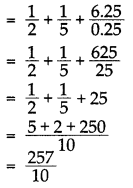(ii) $$\frac{2}{5}-\frac{1}{4}$$ + (8.1 × 2.7) ÷ 0.09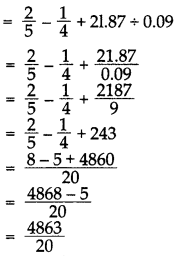(iii) 1.44 × (144 ÷ 12) – 0.225 + 3.276
= 1.44 × $$\frac{144}{12}$$ – 0.225 + 3.276
= 1.44 × 12 – 0.225 + 3.276
= 17.28 + (3.276 – 0.225)
= 17.28 + 3.051
= 17.280 + 3.051 = 20.331
= $$\frac{20331}{1000}$$

(iv) $$\frac{1}{7}$$ × 0.049 + $$\frac{3}{8}-\frac{7}{20}$$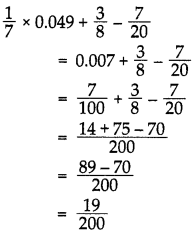(v) 5 × 0.16 – 0.52 + 8.263
5 × 0.16 – 0.52 + 8.263
= 0.80 – 0.52 + 8.263
= 0.800 – 0.520 + 8.263
= (0.800 + 8.263) – 0.520
= 9.063 – 0.520
= 8.543

(vi) $$\frac{2}{5} \times \frac{3}{4}+\frac{1}{25} \times \frac{1}{2}-\frac{2}{10} \times \frac{1}{5}$$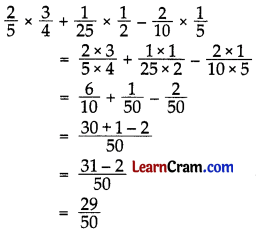### DAV Class 7 Maths Chapter 1 Value Based Questions

Question 1.
Megha bought a book for ₹ 112$$\frac{1}{2}$$ from a shop. She gave 500 rupee note to shopkeeper and go the balance back. But, she realised that the shopkeeper had given her ₹ 72 extra. Megha returned the extra money and had a feeling of great satisfaction.
(i) How much money had the shopkeeper returned to Megha?
Price of book = ₹ 112$$\frac{1}{2}$$
= ₹ $$\frac{225}{2}$$
= ₹ 112.5
Money that was given by Megha to shopkeeper = ₹ 500
∴ Balance = ₹ 500 – ₹ 112.5
= ₹ 387.5
Now, money that had been returned to megha by the shopkeeper = ₹ 387.5 + ₹ 72
= ₹ 459.5
= ₹ 459

(ii) What values did Megha exhibit in the above situation?
Values: Megha is a honest girl. She showed honesty by returning th extra money to the shopkeeper.Question 2.
Raman had to cover a distance of 30 km to reach his grandmother’s house. He covered 11.25 km by bus, 7.083 km by auto and rest by foot.
(i) How much distance did Roman cover by foot?